# SAT II Math II : SAT Subject Test in Math II

## Example Questions

### Example Question #1 : Median

Determine the median of the numbers: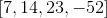Possible Answers: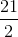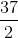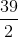Correct answer:Explanation:

Order the data set from least to greatest.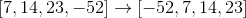The median is the average of the two central numbers for an even amount of numbers in the data set.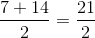The answer is:### Example Question #1 : Median

Determine the median of the following numbers: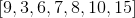Possible Answers: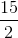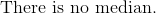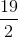Correct answer:Explanation:

Organize the numbers from least to greatest.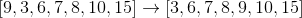The median of an odd set of numbers is the central number.

The answer is:### Example Question #1 : Mode

Find the mode of the following data set: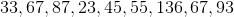Possible Answers: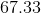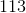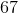Correct answer:Explanation:

Find the mode of the following data set:The mode will be the most repeated number. To find it, simply see which number appears most.

In this case, we have two 67's, and only 1 of each other term.

Therefore, our mode is 67.

### Example Question #2 : Mode

Determine the mode of the following numbers: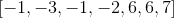Possible Answers: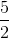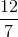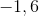Correct answer:Explanation:

The mode is defined as the number or numbers that has the highest frequency in the data set.

The numbersappear in the data set twice, while the other numbers appear once.

This means that the mode is:### Example Question #3 : Mode

Calculate the mode: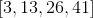Possible Answers: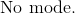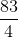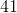Correct answer:Explanation:

The definition of mode is the number or numbers that have the highest frequency in a data set.

There is only one of each number given in the data set.

This means that there is no mode.

The answer is:### Example Question #4 : Mode

Determine the mode: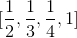Possible Answers: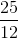Correct answer:Explanation:

The mode includes all numbers that have more than one frequency.  Since every number only occurs once in the set of data, there is no mode.

The answer is:### Example Question #1 : Mode

Determine the mode of the numbers: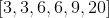Possible Answers: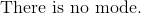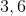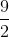Correct answer:Explanation:

The mode includes all numbers that have the highest frequency.

The numbers three and six occur twice, while the other numbers appear once.

The answer is:### Example Question #1 : Range

Find the range of the following data set:Possible Answers:Correct answer:Explanation:

Find the range of the following data set:To find the range, find the difference between the largest and smallest values.

Largest: 136

Smallest:23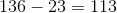So our range is 113

### Example Question #1 : Range

Determine the range of the numbers.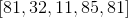Possible Answers: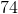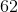Correct answer:Explanation:

The range of the numbers is the difference between the largest and smallest numbers.

The largest number is 85.

The smallest number is 11.

Subtract both numbers.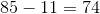The answer is:### Example Question #3 : Range

Determine the range of the numbers: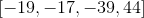Possible Answers: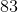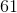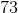Correct answer:Explanation:

To find the range, subtract the highest with the lowest number provided in the set of numbers.

The highest number is 44.

The smallest number is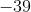.

Subtract both quantities.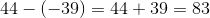The answer is:### All SAT II Math II Resources